# The Bayesian Paradigm - Part 2¶

Sept. 6, 2018 $\newcommand{\bs}{\boldsymbol}$ $\newcommand{\argmin}{\underset{\bs{#1}}{\text{arg min}}\,}$ $\newcommand{\argmax}{\underset{\bs{#1}}{\text{arg max}}\,}$ $\newcommand{\tr}{^{\top}}$ $\newcommand{\norm}{\left|\left|\,#1\,\right|\right|}$ $\newcommand{\given}{\,|\,}$

In my previous post, I used the exponential distribution as the modeled distribution. In this post, I will repeat the same process, but using the Poisson distribution. In addition, I will dive more deeply into Bayesian methods. We expect similar results due to the exponential-Poisson relationship, which states that if $X \sim \text{Exponential}(1/\lambda)$, for any $t>0$, we have

$$P(X\leq t) = P(Y\geq 1),$$

where $Y\sim \text{Poisson}(\lambda t)$. Let's start with another motivating example.

Example Yesterday, I stayed near Sikes Hall's bus stop observing the traffic. I counted 2 busses passing by the bus stop during my stay. What is a reasonable probability model for $\lambda$, the expected number of busses that arrives at the bus stop per hour?

Let $Y$ denote the number of bus that pass by a bus stop in an hour. It is reasonable to assume that $Y$ follows a Poisson distribution with rate parameter $\lambda$. So the probability mass function is

$$p_{\text{model}}(y; \lambda) = \frac{e^{-\lambda}\lambda^y}{y!} I(y\in \{0, 1,...\}). \tag{1}$$

Let's plot the probability mass function of the Poisson distribution for various values of $\lambda$.

In :
options(repr.plot.width=6, repr.plot.height=4)

par(mfrow=c(2,2), mar = c(4, 4, 1, 1))
t <- seq(0, 12, by=1)
lambda <- c(1/2, 2/3, 1, 2)

for (i in 1:4) {
plot(t, dpois(t, lambda[i]),
main=bquote(paste(lambda, "=", .(round(lambda[[i]], 2)))),
t='b', col='blue',
ylab='probability mass', ylim=c(0, 0.6))
}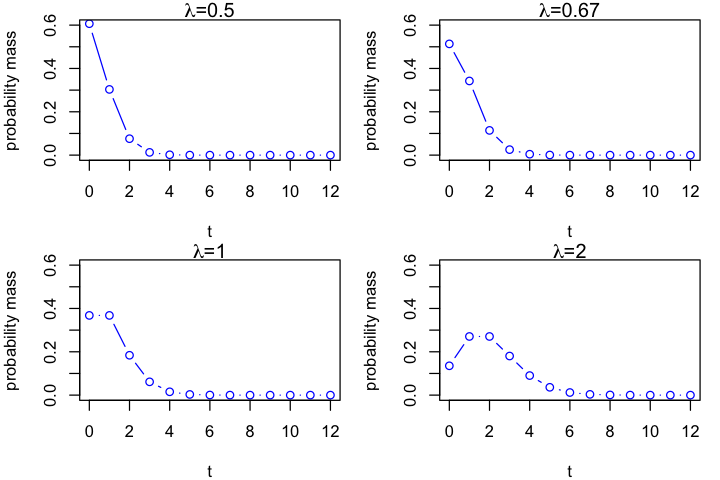Alternatively, we can use ggplot2 to produce a more fancy plot.

In :
library(dplyr)
library(data.table)
library(ggplot2)
library(reshape2) # for the melt() function

In :
options(repr.plot.width=4, repr.plot.height=3)

# Creating four different arrays of values
t <- seq(0, 12, by=1)
df.1 <- dpois(t, 2)
df.2 <- dpois(t, 1)
df.3 <- dpois(t, 2/3)
df.4 <- dpois(t, 0.5)

# Combine them into a single data table
data <- cbind(t, df.1, df.2, df.3, df.4) %>% as.data.table()
names(data) <- c('t', '2', '1', '2/3', '1/2')

# The melt function is nice for plotting as it converts a data frame into a
# single array with two additional columns "value" and "variable"
data_long <- melt(data, id="t")
ggplot(data_long, aes(x=t, y=value, color=variable)) +
geom_line() + geom_point() +
labs(title = "The Poisson Distribution", x = expression(Y), y = "Probability Mass") +
guides(color=guide_legend(title=expression(lambda)))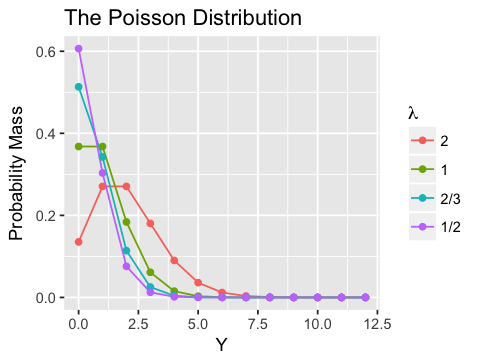From these plots we see that as the value of $\lambda$ increases, the probability mass gets more "normal like". The $\lambda$ is also referred to as the arrival intensity of a Poisson process.

## Frequentist Approach¶

From my earlier post, the most popular frequentist apporach is by minimizing the cross entropy between the empirical distribution and the modeled distribution defined in (1). In this case, the empirical distribution is just $\widehat{p}(2)=1$. Hence, we want to minimize

\begin{aligned} H(\widehat{p}, p_{\text{model}}) &= E_{t\sim \widehat{p}} -\log p_{\text{model}}(t; \theta) \\ &= -\log\left(\frac{e^{-\lambda} \lambda^2}{2!}\right) \\ &= \lambda - 2\log \lambda + \log 2 \end{aligned}

Differentiating the cross entropy and setting it equal to 0 we have the maximum likelihood estimator:

$$\widehat{\lambda}_{\text{MLE}} = 2$$

In general, if we have data $y_1, y_2,..., y_n$, and assuming that they are independent and identically distributed, so that the empirical distribution is defined as $\widehat{p}(y_i)=\frac{1}{n}, i=1,..., n$, the cross entropy is

\begin{aligned} H(\widehat{p}, p_{\text{model}}) &= E_{t\sim \widehat{p}} -\log p_{\text{model}}(t; \theta) \\ &= \frac{1}{n}\sum_{i=1}^n -\log\left(\frac{e^{-\lambda} \lambda^{y_i}}{y_i!}\right) \\ &=\frac{1}{n}\sum_{i=1}^n \left(\lambda - y_i\log \lambda + \log y_i\right) \\ &= \lambda - \log \lambda \left(\frac{1}{n}\sum_{i=1}^n y_i\right) + \log y_i \end{aligned}

Differentiating the above with respect to $\lambda$ and set it to 0, we have

$$1-\frac{1}{\lambda}\left(\frac{1}{n} \sum_{i=1}^n y_i \right) \overset{\text{set}}{=} 0$$

So the maximum likelihood estimator is $$\widehat{\lambda}_{\text{MLE}} = \frac{1}{n} \sum_{i=1}^n y_i.\tag{2}$$

One of the most powerful weapon in a frequentist's arsenal is the Central Limit Theorem (CLT), which states that the sum of many independent random variables approximately follows a normal distribution. We state it more formally below.

Central Limit Theorem Let $X_1, X_2,...$ be a sequence of iid random variables with finite first and second moments, then

$$\sqrt{n}\,(\bar{X}_n - \mu) \overset{d}{\to} N(0, \sigma^2).$$

Let's apply the CLT to (2). First note that the MLE is nothing more than the sample mean of $Y$. Also since each $Y_i$ is Poisson distributed with both mean and variance equal to $\lambda$, the Central Limit Theorem gives

$$\sqrt{n}\,\left(\widehat{\lambda}_{\text{MLE}} - \lambda \right) \overset{d}{\to} N\left(0, \lambda\right).$$

This means as $n$ gets "large", we have that

$$\widehat{\lambda}_{\text{MLE}} \overset{\text{approx}}{\sim} N\left(\lambda, \frac{\lambda}{n} \right).$$

Since the variances goes to 0, $\widehat{\theta}_{\text{MLE}}$ is a consistent estimator.

Using the asymptotic approximation, we can find the frequentist's greatest weapon, the $100(1-\alpha)\%$ confidence interval, which is given by

$$\widehat{\lambda}_{\text{MLE}} \pm z_{1-\alpha/2} \sqrt{\frac{\widehat{\lambda}_{\text{MLE}}}{n}}$$

where $z_{1-\alpha/2}$ is the critical value corresponding to the $100(1-\alpha/2)$th percentile of the standard normal density.

Since $n=1$ in our example, we cannot use the CLT. But we can simulate many $\text{Poisson}(2)$ random variables, and find a confidence interval numerically.

In :
# create 100000 randomly generated estimators
set.seed(1)
rpois(100000, 2) -> sample

In :
# a function that returns the percentage of sample points in an interval around a point estimate
sample.percentage <- function(sample, lambda=2, h=0.1) {
lambda.lower <- lambda - h
lambda.upper <- lambda + h
percentage <- mean(sample > lambda.lower & sample < lambda.upper)
return(percentage)
}

In :
# recursively find the confidence interval based on alpha
sample.CI <- function(sample, lambda=2, h=0.1, alpha=0.05, tolerance = 0.01) {
percentage <- sample.percentage(sample, lambda, h)
if (percentage < 1 - alpha - tolerance) {
h <- h + 0.01
sample.CI(sample, h=h)
} else if (percentage > 1 - alpha + tolerance) {
h <- h - 0.01
sample.CI(sample, h=h)
} else {
return(c(max(0, lambda-h), lambda+h, percentage))
}
}

In :
print(sample.CI(sample))

 0.00000 4.00000 0.94697


To find the exact value of $h$, we minimizing the $L2$ loss function:

$$L(p, 0.95) = (\text{sample.percentage}(h)-0.95)^2$$

In :
# Define the L2 loss function
sample.loss <- function(sample, lambda=2, h=0.1) {
(sample.percentage(sample, lambda, h)-0.95)^2
}

# minimize the L2 loss function
h.optimal <- optimize(sample.loss, interval=c(0,12), sample=sample, lambda=2, maximum = F)$minimum print(paste0("The 95% confidence interval is ", "(", max(0, 2-h.optimal), ", ", 2+h.optimal, ")."))   "The 95% confidence interval is (0, 4.41956639327475)."  So based on only one data point, the 95% confidence interval is found to be apprximately$[0, 4.4]$. The biggest downside of the frequentist confidence interval is that it is difficult to interpret. One is tempted to think that there is a 95% probability that the true value of$\lambda$lies in the interval$[0, 4]$. This is incorrect since the frequentist paradigm deems$\lambda$to be a fixed parameter, so it is meaningless to mention such probability. Instead, we interpret it this way: If we repeat the chance experiment many times (hence the name "frequentist"), we are expected to see that about 95% of the confidence intervals will cover the true value$\lambda$. ## Bayesian Approach¶ Denote by$\bs{y}the data that we observed, the Bayesian approach relies on the Bayes' Theorem: \begin{aligned} p(\lambda \given \bs{y}) &= \frac{p(\lambda)p(\bs{y}\given \lambda)}{p(\bs{y})} \\ &\propto p(\lambda)p(\bs{y}\given \lambda) \end{aligned} \tag{3} Herep(\lambda)$is called the prior distribution. It represents our belief about$\lambda$prior to seeing the data$\bs{y}$. The conditional distribution$p(\lambda \given \bs{y})$is called the posterior distribution. It represents our updated belief about$\lambda$after observing the data$\bs{y}$. As mentioned in my previous post, I know that the busses travel to and fro between two locations, and the maximum number of buses running simultaneously is 6. Below is a screenshot of the live map of the busses. At the time of taking the screenshot, there were four busses running simultaneously.source: http://www.catbus.com/ Same as before, we consider the best case scenario: 1. You see ALL 6 busses passing by a bus stop. 2. You wait at the bus stop for at most one hour. 3. You see 6 busses returning. 4. Since it takes one hour for the bus to travel from one end of the route to the other, you conclude that the maximum number of busses one can observe in a single hour is 12. How do we choose the prior distribution$p(\lambda)$? For ease of computation, we often use the conjugate prior. Definition Consider the class of prior distributions$p(\lambda) \in \mathcal{P}$. We say that the class is conjugate for the modeled distribution$p(y \given \lambda)$if$p(\lambda)\in \mathcal{P}$implies$p(\lambda \given y)\in \mathcal{P}$for all$p(\theta)\in \mathcal{P}$and data$y$. It turns out that the conjugate prior for a Poisson model is the gamma distribution. This can be derived using the definition. Suppose$p(y\given \lambda) \sim \text{Poisson}(\lambda)$and$p(\lambda) \sim \text{gamma}(\alpha, \beta)$where$\beta$is the scale parameter. Given a set of iid data points$\bs{y} = (y_1, y_2,..., y_n)', the posterior density is \begin{aligned} p(\lambda \given \bs{y}) &\propto p(\lambda) p(\bs{y}\given \lambda) \\ &= p(\lambda) \prod_{i=1}^n p(y_i \given \lambda) \\ &= \left(\frac{1}{\Gamma(\alpha) \beta^{\lambda}} \lambda^{\alpha-1} e^{-\frac{\lambda}{\beta}}\right) \left( \prod_{i=1}^n \frac{e^{-\lambda} \lambda^{y_i}}{y_i!}\right) \\ &\propto \frac{1}{\beta^{\lambda}} \lambda^{\alpha + \sum y_i -1} e^{-(1/\beta+n)\lambda} \end{aligned} Note that this is precisely the kernel of the general form of gamma distribution, $$p(\lambda; \alpha, \beta) = \frac{1}{\Gamma(\alpha) \beta^{\lambda}} \lambda^{\alpha-1} e^{-\frac{\lambda}{\beta}}.$$ We conclude that the posterior distribution belongs to the gamma familiy with parameters \begin{aligned} \alpha_{\text{post}} &:= \alpha + \sum_{i=1}^n y_i \\ \beta_{\text{post}} &:= \frac{1}{1/\beta +n} \end{aligned} \tag{4} The awesome benefit of using a conjugate prior is that the posterior mean can be easily computed as\alpha \beta$and the variance$\alpha \beta^2$. Based on the screenshot (not the data), it is reasonable to let$\alpha \beta =4$. Below is a plot for various$\alpha$and$\beta$values with$\alpha\beta=4$. In : options(repr.plot.width=6, repr.plot.height=4) par(mfrow=c(2,2), mar = c(4, 4, 1, 1)) lambda <- seq(0, 12, by=0.1) alpha <- c(1, 2, 4, 8) beta <- 4 / alpha for (i in 1:4) { plot(lambda, dgamma(lambda, alpha[[i]], scale=beta[[i]]), main= bquote(paste(alpha,"=", .(alpha[[i]]))), t='l', col='blue', xlab='', ylab='density', ylim=c(0, 0.4)) }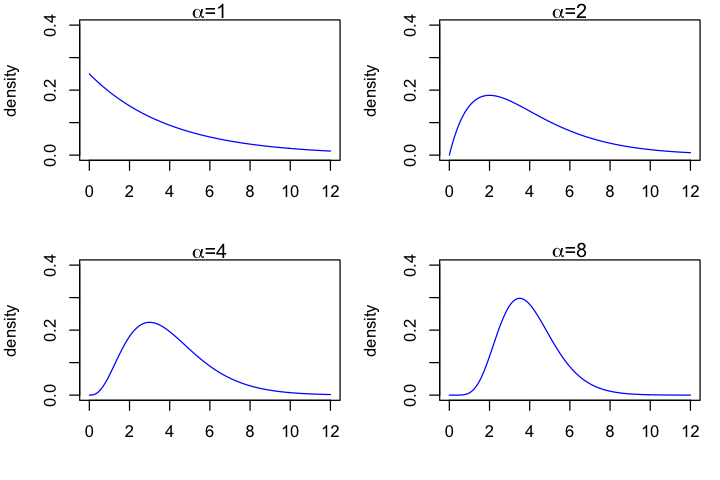It looks like that$\alpha=4$best captures my prior belief about$\lambda$. Hence we choose$\text{gamma}(4,1)$as the prior distribution. Using (4) we can immediately conclude that the posterior distribution follows the$\text{gamma}(6, 0.5)$distribution, with mean equal to 3 and variance equal to 1.5. (Compare this to the prior mean of 4 and variance of 4). Let's visualize it. In : t <- seq(0, 12, by=0.1) prior <- dgamma(t, 4, scale=1) post <- dgamma(t, 6, scale=0.5) data <- cbind(t, prior, post) %>% as.data.table() names(data) <- c('t', 'prior', 'posterior') ggplot(data) + geom_line(aes(x=t, y=prior, color="Prior"))+ geom_line(aes(x=t, y=posterior, color="Posterior")) + labs(title = "Bayesian Updating", x = bquote(lambda), y = "density")+ guides(color=guide_legend(title="Distributions"))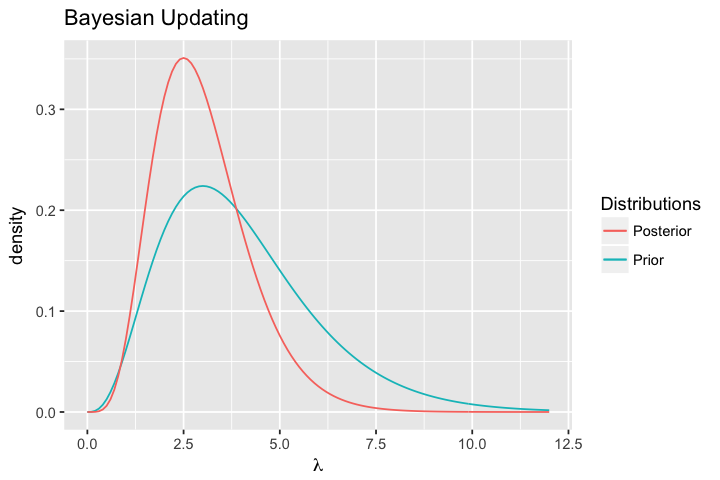The amazing thing here is that the point estimate$3$is not so different from the estimate we obtained from the previous post using the exponential distribution as our model. What about the confidence interval? Here is a definition. Definition The Bayesian credible interval,$[l(\bs{y}), u(\bs{y})]$, based on the observed data$\bs{y}$is an interval that has 95% coverage of$\lambda$, i.e., $$P\left(l(\bs{y}) < \lambda < u(\bs{y}) \given \bs{y}\right) = 0.95.$$ The easiest approach to constructing a$100(1-\alpha)\%$credible intervals is by using the equal-tailed method. The steps are summarized below. 1. First let$\alpha^* = \alpha/2$. 2. Find the values$\lambda^*$and$\lambda^{**}such that \begin{aligned} P(\lambda < \lambda^*) &= \alpha^* \\ P(\lambda > \lambda^{**}) &= \alpha^* \end{aligned} 3. The resulting interval[\lambda^*, \lambda^{**}]is the equal-tailed Bayesian credible interval. To find the equal-tailed credible interval for our problem, we solve \begin{aligned} \int_0^{\lambda^*} p(\lambda \given \bs{y})\, d\lambda &= 0.025 \\ \int_{\lambda^{**}}^{\infty} p(\lambda \given \bs{y})\, d\lambda &= 0.025. \end{aligned} In R this can be easily accomplished using the qgamma function, which returns the quantiles of a gamma distribution. In : # define a function that returns the equal-tailed credible interval DT.gamma <- function(data, alpha, beta, significance) { alpha.post <- alpha + sum(data) beta.post <- 1/(1/beta + length(data)) dt.lower <- qgamma(significance/2, alpha.post, scale=beta.post) dt.upper <- qgamma(1-significance/2, alpha.post, scale=beta.post) print(paste0("The ", 100*significance, "% equal-tailed credible interval is ", "(", dt.lower, ", ", dt.upper, ").")) return(c(dt.lower, dt.upper)) }  In : DT.gamma(data=c(2), alpha=4, beta=1, 0.05)   "The 5% equal-tailed credible interval is (1.10094712674543, 5.83416603966133)."  1. 1.10094712674543 2. 5.83416603966133 But is this the best interval we can construct? By the frequentist preference, we want the credible interval to be as narrow as possible. This can be accomplished using the highest posterior density (HPD) region. Definition A100(1-\alpha)\%$HPD region consists a subset of the support of$\theta$, denoted by$s(\bs{t})$, such that 1.$P(\theta \in s(\bs{t}) \given \bs{T}=\bs{t}) = 1-\alpha$2. If$\theta^* \in s(\bs{t})$and$\theta^{**} \not\in s(\bs{t})$, then$p(\theta^* \given \bs{t}) > p(\theta^{**} \given \bs{t})$. To illustrate the concept of HPD, we generate a bimodal mixture Guassian distribution: $$0.4\times N(-1, .5^2) + 0.6\times N(3, 0.8^2)$$ In : par(mar = c(4, 4, 1, 1)) t <- seq(-3, 6, by=0.1) # mixed Guassian density dist <- function(t){0.4*dnorm(t, -1, 0.5) + 0.6*dnorm(t, 3, 0.8)} # the mixed Guassian minus a constant (for root finding) dist2 <- function(t){0.4*dnorm(t, -1, 0.5) + 0.6*dnorm(t, 3, 0.8) - 0.15} # finding the intercept between y=0.15 and the mixed density x1 <- uniroot(f=dist2, lower=-3, upper=-1)$root
x2 <- uniroot(f=dist2, lower=-1, upper=1)$root x3 <- uniroot(f=dist2, lower=1, upper=3)$root
x4 <- uniroot(f=dist2, lower=3, upper=5)$root # creating a plot with shaded region left.x <- c(x1, seq(x1, x2, 0.01), x2) left.y <- c(0, dist(seq(x1, x2, 0.01)), 0) right.x <- c(x3, seq(x3, x4, 0.01), x4) right.y <- c(0, dist(seq(x3, x4, 0.01)), 0) plot(t, dist(t), t='l', ylab='density') polygon(left.x, left.y, col="red") polygon(right.x, right.y, col="red") abline(h=0.15, lty=2)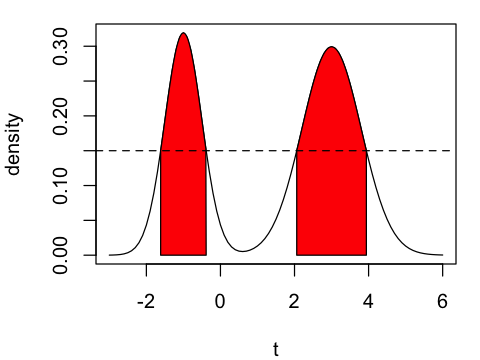From the plot above, the$x$-values for the sharded region, whose area is$100(1-\alpha)\%$, corresponds to the HPD intervals. It can be proven that the HPD interval is the shortest amongst all$100(1-\alpha)\%$credible intervals. The algorithm of finding the HPD interval for an unimodal distribution is easy to design. Algorithm (Finding HPD interval) • Find the mode$\tilde{\theta}$of the posterior density. • Construct a relative density by dividing the posterior density by its maximum value: $$r(\theta) := \frac{p(\theta \given t)}{p(\tilde{\theta} \given t)}.$$ • Choose a height$h\in (0, 1)$. Find the values$a < \tilde{\theta}$such that$r(a) = h$. Find the value$b > \tilde{\theta}$such that$r(b) = h$. • Calculate$P(a<\theta < b \given T=t)$. • Adjust the value of$h$until the probability converges to 0.95. To implement this altorithm in R, we first create a function that returns the HPD interval and the associated probability with a fixed value of$h \in (0, 1)$. In : # function that calculates the HPD interval given a value of h HPD.gamma.h <- function(data, alpha=4, beta=1, h=0.5, left=0, right=12, plot=T) { alpha.post <- alpha + sum(data) beta.post <- 1/(1/beta + length(data)) density.post <- function(x){dgamma(x, alpha.post, scale=beta.post)} # finding the mode using the optimization function mode.x <- optimize(density.post, interval=c(left, right), maximum=TRUE)$maximum
mode.y <- density.post(mode.x)

# creating a normalized distributions
r <- function(x){density.post(x)/mode.y}
r2 <- function(x){r(x) - h}

# finding the interval
a <- uniroot(f = r2, lower=left, upper=mode.x)$root b <- uniroot(f = r2, lower=mode.x, upper=right)$root

# calculating the coverage probability
coverage <- integrate(density.post, a, b)$value if (plot) { options(repr.plot.width=4, repr.plot.height=3) par(mar = c(4, 4, 1, 1)) t <- seq(0, 12, by=0.1) # creating a plot with shaded region left.x <- c(a, seq(a, b, 0.01), b) left.y <- c(0, r(seq(a, b, 0.01)), 0) plot(t, r(t), t='l', ylab='posterior density') polygon(left.x, left.y, col="red") abline(h=h, lty=2) title(bquote(paste("P(", .(round(a, 2))," < ", lambda, " < ", .(round(b,2)), " | " , y, ") = ", .(round(coverage, 2))))) } return(c(a, b, coverage)) }  In : HPD.gamma.h(data=c(2))  1. 1.40377392352548 2. 4.05687211039147 3. 0.753192008239257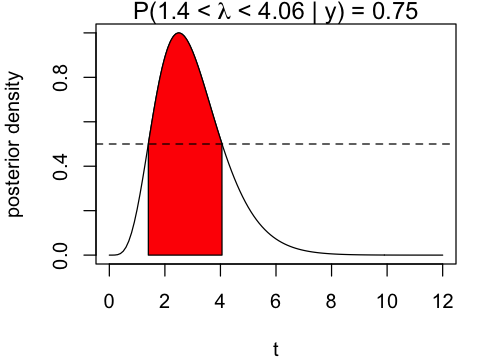Similar as before, to find the exact value of$h$value, we minimizing the$L2$loss function: $$L(p, 0.95) = (\text{HPD.gamma.h}(h)-0.95)^2$$ In : # Define the L2 loss function HPD.loss <- function(h, data, alpha, beta) { coverage <- HPD.gamma.h(data, alpha, beta, h, plot=F) (coverage-0.95)^2 } h.optimal <- optimize(HPD.loss, interval=c(0,1), data=c(2), alpha=4, beta=1, maximum = F)$minimum
HPD.gamma.h(data=c(2), h=h.optimal)

1. 0.879032689432756
2. 5.43221017363795
3. 0.94999965158167Comparing this to the equal-tailed interval $[1.1, 5.8]$, the HPD interval $[0.88, 5.43]$ is indeed shorter!

So the Bayesian HPD credible interval is found to be $[1.1, 5.8]$. Comparing that with the equal-tailed credible interval of $[0.88, 5.43]$, we see that the HPD interval has a shorter width. Interestingly, unlike in my previous post where we used the exponential model, the Bayesian credible interval is very similar to the frequentist's estimate of $[0, 4.4]$.

## Combined Model¶

Now we are ready to define a combined model for a data set $\bs{y}=(y_1, y_2,..., y_n)$.

In :
model.Poisson <- function(data, significance=0.05, alpha=4, beta=1, h=0.5, left=0, right=12, frequentist=T, plot=T){
if (frequentist) {
lambda.MLE <- mean(data)
SE <- qnorm(1-0.05/2)*sqrt(lambda.MLE/length(data))
return(c(lambda.MLE - SE, lambda.MLE + SE))
} else {
h.optimal <- optimize(HPD.loss, interval=c(0,1), data=data, alpha=alpha, beta=beta, maximum = F)$minimum HPD.gamma.h(data=data, h=h.optimal) } }  Can't wait to test it out! Let's generate 50 observations from the Poisson distribution with true mean$\lambda = 3\$.

In :
set.seed(1)
mydata <- rpois(50, 3)
print(mydata)

  2 2 3 5 2 5 6 4 3 1 2 1 4 2 4 3 4 8 2 4 6 2 4 1 2 2 0 2 5 2 3 3 3 1 5 4 4 1
 4 2 5 3 4 3 3 4 0 3 4 4

In :
model.Poisson(mydata)

1. 2.63040115358458
2. 3.60959884641542
In :
model.Poisson(mydata, frequentist=F)

1. 2.65760297268835
2. 3.62794425079901
3. 0.949999390183714In :
model.Poisson(mydata, frequentist=F, alpha=2, beta=2)

1. 2.65759975869364
2. 3.62794820559001
3. 0.950001080474834In :
model.Poisson(mydata, frequentist=F, alpha=8, beta=0.5)

1. 2.65760909976455
2. 3.62793671150417
3. 0.949996167727502We see that for large data sets, the frequentist and Poisson approaches produce very similar results regardless of the choice of the prior distribution.

## Bayesian Updating¶

Finally, I am curious to see how the posterior density evolves over time by sequentially feeding my data into the algorithm.

In :
options(repr.plot.width=6, repr.plot.height=4)

lambda <- seq(1, 6, by=0.05)
prior <- dgamma(lambda, 4, scale=1)

# sequentially truncating my data
mydata.epoch1 <- mydata[1:10]
mydata.epoch2 <- mydata[1:20]
mydata.epoch3 <- mydata[1:30]
mydata.epoch4 <- mydata[1:40]
mydata.epoch5 <- mydata[1:50]

# posterior distribution in epochs
post.epoch1 <- dgamma(lambda, 4 + sum(mydata.epoch1), scale=1/(1+10))
post.epoch2 <- dgamma(lambda, 4 + sum(mydata.epoch2), scale=1/(1+20))
post.epoch3 <- dgamma(lambda, 4 + sum(mydata.epoch3), scale=1/(1+30))
post.epoch4 <- dgamma(lambda, 4 + sum(mydata.epoch4), scale=1/(1+40))
post.epoch5 <- dgamma(lambda, 4 + sum(mydata.epoch5), scale=1/(1+50))

data <- cbind(lambda, prior, post.epoch1, post.epoch2, post.epoch3, post.epoch4, post.epoch5) %>% as.data.table()
names(data) <- c('lambda', 'prior', 'epoch1', 'epoch2', 'epoch3', 'epoch4', 'epoch5')

ggplot(data) +
geom_line(aes(x=lambda, y=prior, color="Prior"))+
geom_line(aes(x=lambda, y=epoch1, color="Epoch 1")) +
geom_line(aes(x=lambda, y=epoch2, color="Epoch 2")) +
geom_line(aes(x=lambda, y=epoch3, color="Epoch 3")) +
geom_line(aes(x=lambda, y=epoch4, color="Epoch 4")) +
geom_line(aes(x=lambda, y=epoch5, color="Epoch 5")) +
labs(title = "Bayesian Updating", x = bquote(lambda), y = "density")+
guides(color=guide_legend(title="Distributions"))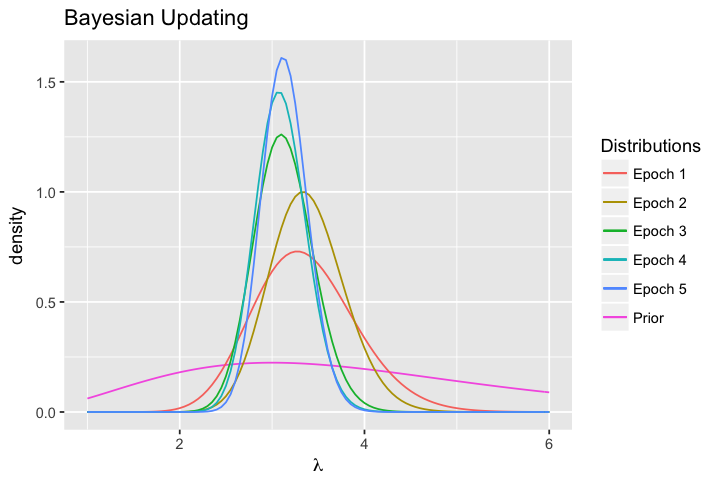Conclusion: Bayesian statistics is beautiful.